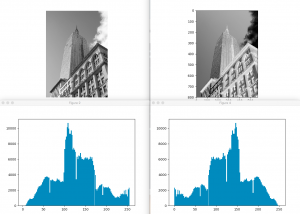# 第一章 基本图像处理

## 第二节 Matplotlib

### 直方图

``````from PIL import Image
from pylab import *

im = array(Image.open('images/empire.jpg').convert('L'))
print im.shape, im.dtype  # (800, 569) uint8

figure()
gray()
axis('equal')
axis('off')
imshow(im)

figure()
h = hist(im.flatten(), 128)
print len(h), h

show()
``````